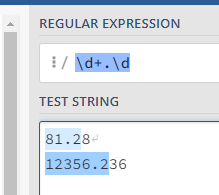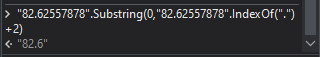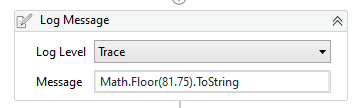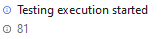# How do i Remove the Digits After Decimal Wihout Round?

Hello

I want the Number Without Rounding …

i.e

I have Number 81.28 and I just want 81.2 without Rounding

I have tried Cdbl and Double Funtion but it Convert it to 81.3

Hello @kishan.savaliya
You can use Regex method

``````System.Text.RegularExpression.Regex.Match(YourString,"\d+.\d").ToString.Trim
``````2 Likes

You can also try with below expression

``````"82.62557878".Substring(0,"82.62557878".IndexOf(".")+2)
``````Regards
Gokul

1 Like

Hi @kishan.savaliya
Try Generic
InputStr.Substring(0,InputStr.split("."c)(0).length+2)

2 Likes

this will fail if more than two decimals there right?

1 Like

Hi,
Try This
cdbl(System.Text.RegularExpressions.Regex.Match(cstr(Str_value),“([0-9]+).([0-9]{1})”).Value)

xaml is attached
round_off.xaml (5.0 KB)

Thanks,
Muthuraj

1 Like

Thanks for letting me know and i have updated the expression @megharajky

2 Likes

Hello. Another method would be with using String.Format

System.String.Format(“{0:0.00}”, number)

1 Like

Use Math.Floor

Math.Floor(81.28) will return 81. Math.Floor(81.75) will still return 81 instead of rounding up.1 Like

Here is another method, which calculates number as a sum of digits:

(CInt(number) + 0.1 * CDbl(CInt(10 * number) - 10 * CInt(number)) + 0.01 * CDbl(CInt(100 * number) - 10 * CInt(10 * number)) ).toString

1 Like

For just 1 decimal this would be:

(CInt(number) + 0.1 * CDbl(CInt(10 * number) - 10 * CInt(number))).toString

1 Like

Sorry that one made a rounding for the one digit.
You may try something else:

(CInt(number) + (Microsoft.VisualBasic.Conversion.Fix(10 * number) - 10 * Microsoft.VisualBasic.Conversion.Fix(number)) / 10).toString